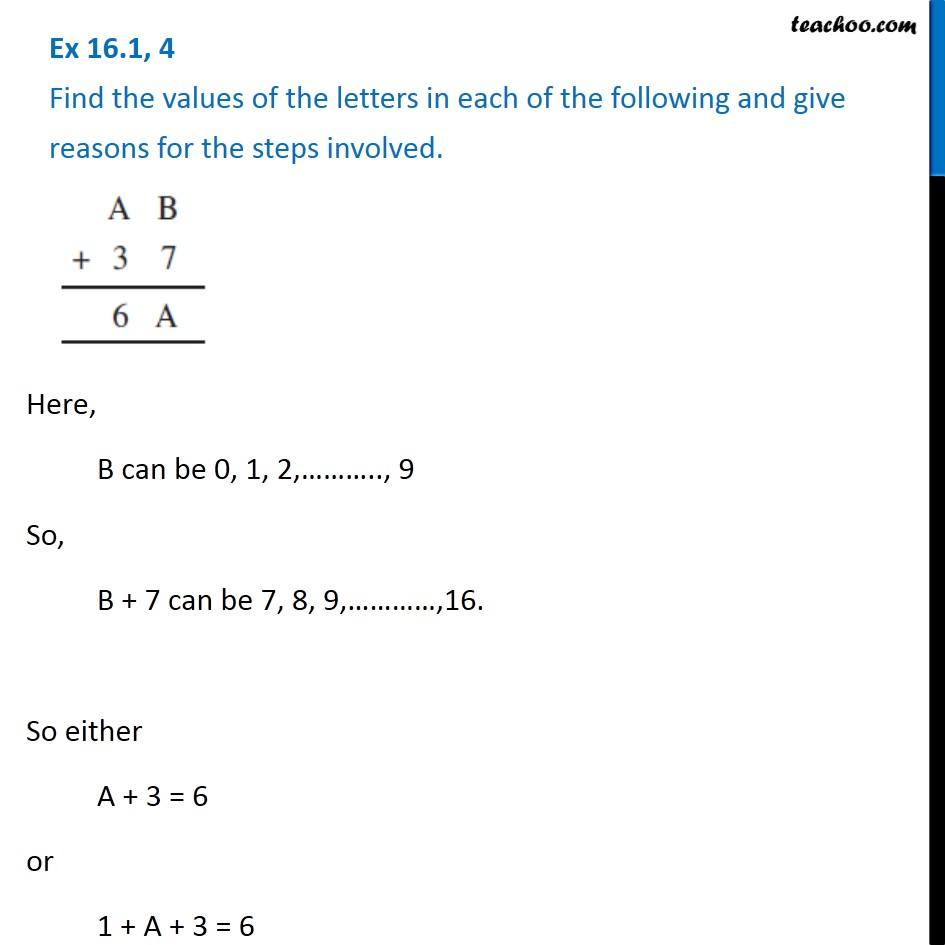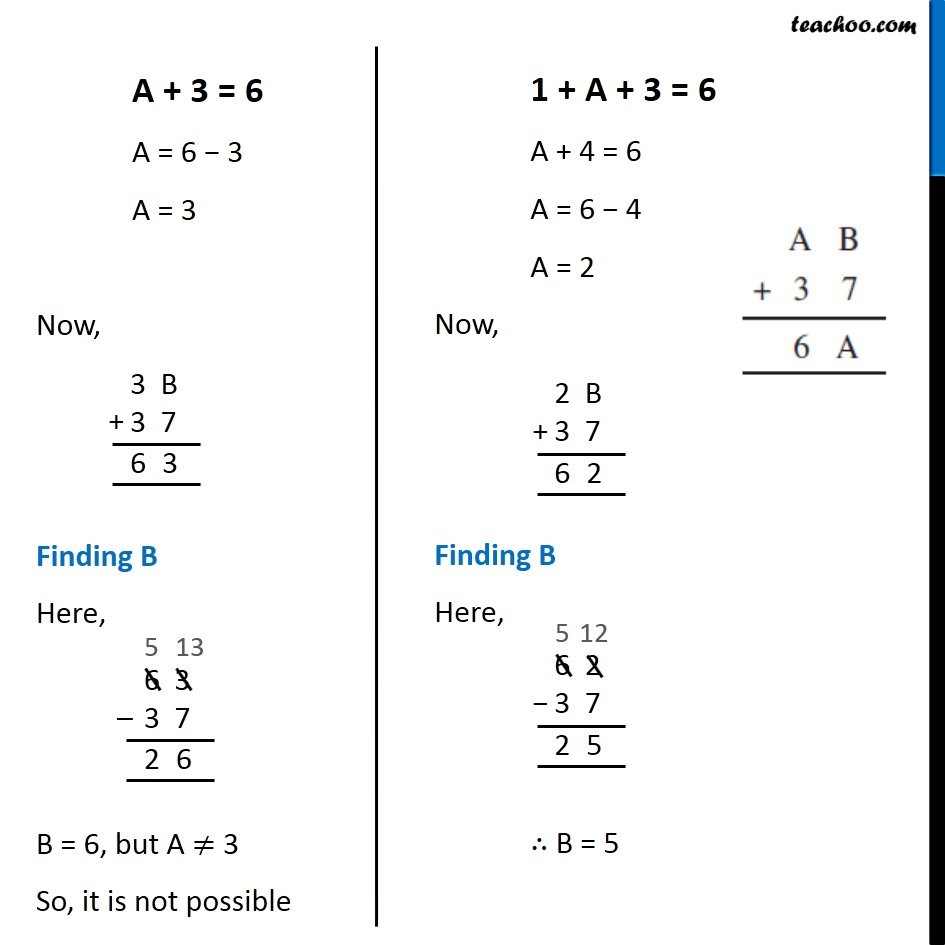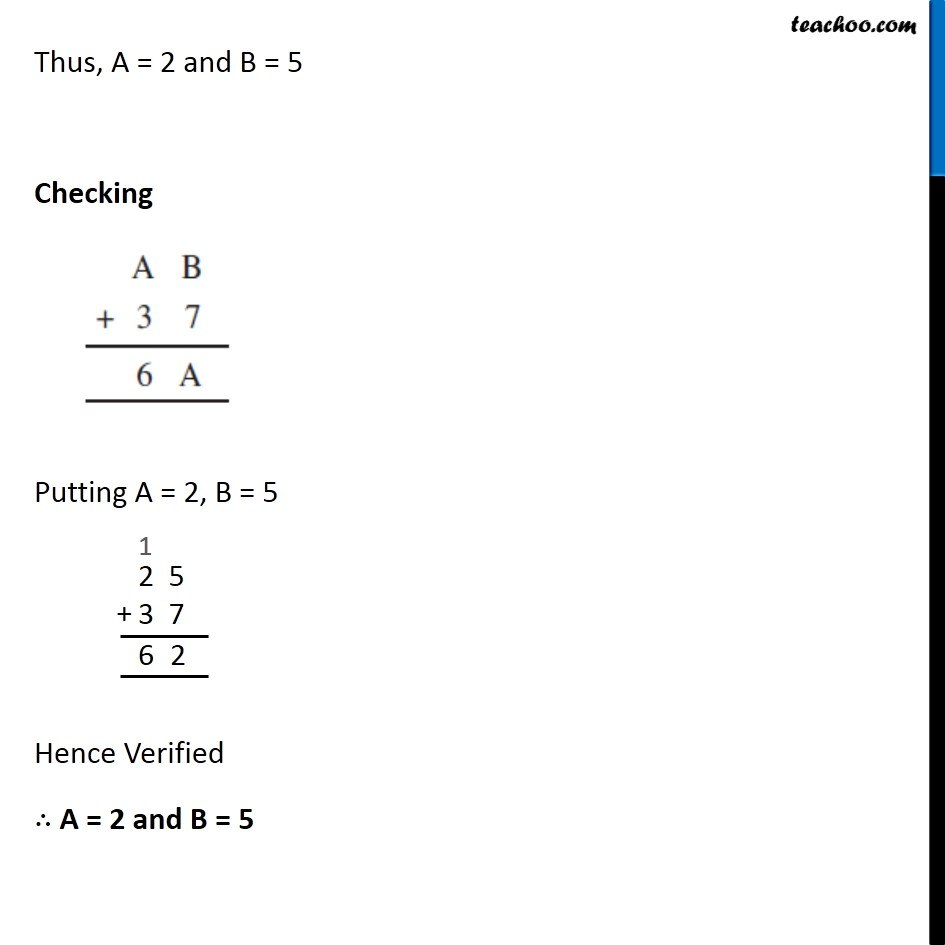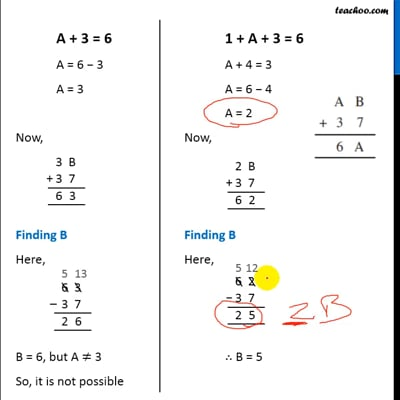Letters for Digits - Addition Questions

Chapter 16 Class 8 Playing with Numbers
Concept wiseThis video is only available for Teachoo black users

Introducing your new favourite teacher - Teachoo Black, at only ₹83 per month

### Transcript

Ex 16.1, 4 Find the values of the letters in each of the following and give reasons for the steps involved. Here, B can be 0, 1, 2,……….., 9 So, B + 7 can be 7, 8, 9,…………,16. So either A + 3 = 6 or 1 + A + 3 = 6 A + 3 = 6 A = 6 − 3 A = 3 Now, Finding B Here, B = 6, but A ≠ 3 So, it is not possible 1 + A + 3 = 6 A + 4 = 6 A = 6 − 4 A = 2 Now, Finding B Here, ∴ B = 5 Thus, A = 2 and B = 5 Checking Putting A = 2, B = 5 Hence Verified ∴ A = 2 and B = 5